# An Introduction to Ohm’s Law and Power

In the previous article, we explored the concepts of voltage and current.

We didn’t however talk about how the two work together. In doing so we’ll need to understand another key concept, resistance. From there we can define Ohm’s Law, which ties all three parameters together.

### Voltage Drives Current

Without voltage no current will flow in a circuit. More formally, without a voltage difference between two points no current will flow.

We understand, for example, that a new battery with a certain voltage between its two terminals has energy that we can use to do work. It took energy to make one terminal of the battery have excess electrons and the other have a deficit. When we put the battery in a circuit, the electrons flow (that is, a current is created), carrying with them a certain amount of energy (e.g. 12 Joules for every coulomb for a 12V battery). When the charges on one terminal are equal to the other, there’s no “motivation” for the electrons to want to go anywhere, and so the current stops.

Incidentally, this really applies to older types of batteries or ones you can make in a home science experiment. A bit later we’ll see why a battery can be dead but still measure almost full voltage.

The key takeaway is that electrons don’t flow without a reason.

As you may have already realized, voltage can exist without current. A 12V battery is still a 12V battery even when it’s not connected to anything. Actually, another term for voltage is electrical potential difference. It’s not as pithy, but the word “potential” tells you that the energy is there whether you’re using it or not.

### Resistance Slows Current

If you have a particular voltage, say from a battery, how do you know how much current will flow? If we connected the two terminals of a battery together with a piece of wire, we’d soon realize the wire was getting hot … fast. The charges from one terminal could travel to the other terminal without any obstacle. The current would surge and we’d quickly either deplete our battery or do damage. We need a way of controlling how much current we want to introduce into our circuit.

The resistor is our way of controlling current. It slows down the rate of charge flow going through it.

The unit of resistance is the ohm, which we’ll define explicitly in a bit. Electrons can’t flow at one rate before the resistor, another rate through the resistor, and another rate after the resistor. This means that if you put a resistor in a circuit, the current through the entire circuit will be the same (this assumes a simple circuit without any parallel branches).

Resistors can be made from several types of material, carbon being the oldest. The atomic organization of atoms like carbon is such that their electrons are not very free to move around. When charges from a current come in to a resistor they bounce around the atoms and lose energy. This bouncing around effectively slows the rate of electrons passing through the resistor, and we therefore see a lowered current. The bouncing around also imparts kinetic energy at the atomic scale to the resistor, which we recognize as heat.

Conductors such as copper have a much different atomic organization than materials such as carbon. The electrons in conductors are relatively free to float around, so when new electrons come rushing in from current in the circuit, it’s easy to displace the electrons the conductor.

Materials that don’t conduct any electricity for any practical use are called insulators. We use insulators such as rubber and plastic to house the conductors to protect us from harm as well as make sure other elements don’t touch the conducting wires and change the nature of the circuit.

### Resistance Lowers Voltage

When the electrons bounce around a resistor heat is given off. This energy comes from the voltage, or Joules/coulomb, carried by our current. So while resistors reduce the current, they also lower the voltage seen by the rest of the circuit at any point after the resistor. The term voltage drop is often used to describe this effect.

If you have a power source of a particular voltage, but elements in your circuit need less voltage, you can put resistors before them to reduce the voltage to operating levels. Most electronics are designed to dissipate as little energy as possible.

Electric heaters, hair dryers, and similar devices on the other hand are designed solely to dissipate electrical energy as heat. It takes a lot of energy, relative to a cell phone for instance, to heat an element to the point of drying hair with a fan. That’s why you might have noticed when your air conditioning unit, electrical heater, or hair dryer turn on your lights dim for an instant. They suck up enough energy to syphon some from other parts of your house.

### Putting It All Together – Ohm’s Law

We now have three concepts, voltage, current, and resistance, and have seen that they all work together in particular ways. The simple mathematical relationship between these three concepts is known as Ohm’s Law, stated below in three different but equivalent ways (I = current, V = voltage, R = resistance):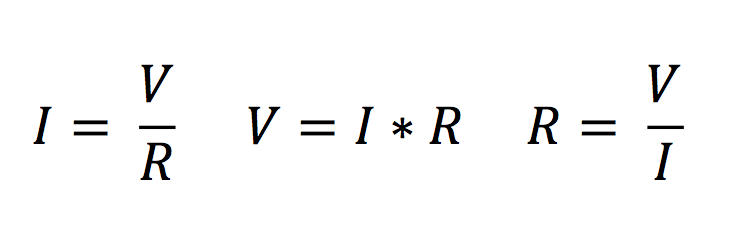We can describe in words the consequences of each form of the equation. The first tells us that increasing the resistance in a circuit for a particular voltage will lower the current. Similarly, increasing the voltage for a particular resistance will increase the current.

The second form of the equation is very useful when you have several resistors in a circuit. Remember that in a simple circuit current has to be the same everywhere. So if we have three different resistors in a circuit each one will dissipate different amounts of energy, even though the current is the same through all of them. Said another way, the voltage drop will be different across each resistor. When we know the value of each resistor and have measured or calculated the current, the second form of the equation can tell us the voltage drop across each.

The last form of the equation is not as commonly used. Resistors are made with markings on them to tell you what their resistance is. A multimeter will also tell you the exact resistance of a resistor. Resistors come with a certain tolerance, usually around 5% or 10%. The third form of the equation can be thought of as a type of definition for a resistor. A resistor is a circuit element that allows one amp to flow when one volt has been applied.

Unfortunately Ohm’s Law is too often given as the definition of what electricity is, leading to confusion, circular definitions, cats and dogs living together, and mass hysteria. With the material we’ve covered so far you should have a much better conceptual understanding of what the three forms of Ohm’s Law actually tell you.

### Power

The term and concept of power too often are used interchangeably with that of energy. We’ve understood and used the word energy in our discussion so far. Energy does work. Without energy, nothing happens. Power is the rate energy is used. The unit of power is the watt, which is defined at consumption or use of 1 Joule every second. With this quantity defined we can see how this relates to electricity: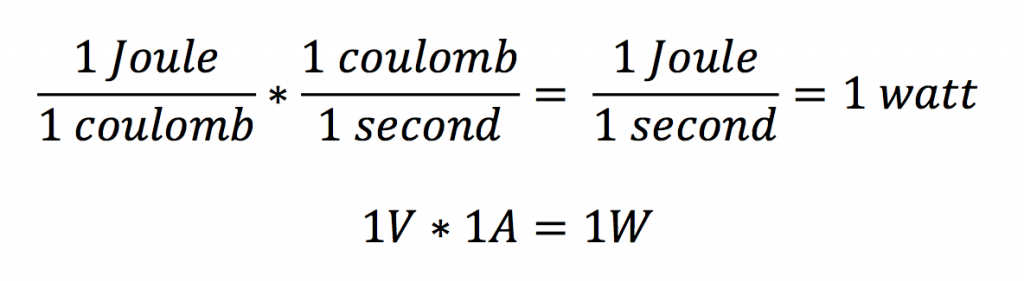It’s nice when things work out like that. We can see why many components like speakers are rated in terms of their wattage. A 200 watt speaker will be capable of handling 200 Joules every second, while a 100 watt speaker will handle 100 Joules a second. We can also see that the 100 watt speaker will handle 200 Joules in 2 seconds. We know the 200 watt speaker will be louder (or at least be louder without distorting) because the rate of energy the speaker can dissipate as sound energy per second is greater.

### Applications and Consequences

With our knowledge of Ohm’s law and power, let’s see how these concepts relate to typical audio situations. Guitar and speaker (1/4” TS) cables      can be of different thickness, or gauge. The American Wire Gauge (AWG) system assigns a number to different thickness of cable. The numbering scheme appears counterintuitive in that cables with higher AWG values are actually thinner cables. The number is not related to how thick the cable is, but to the number of sizing dies the wire needs to be drawn through to reach the desired thickness. Smaller cables need to be drawn through more sizing dies and therefore have a higher AWG value.

Even though copper, the metal usually used in cables, is a conductor, it does have a certain resistance. When the copper wire is thinner in diameter it has more resistance per unit length. Electrons flow more freely when there is more space. Would you rather drive in traffic through five lanes or one? Increasing the length of a cable also increases its resistance. There’s more material for the electrons to pass through, and even with low resistance those electrons will lose energy as they travel, so helping them travel the shortest distance possible will ensure you have to delivered as much energy to your device (e.g. guitar amp) as you can.

The resistance of the guitar amplifier input is so high that the resistance of the cable is often negligible. If the input resistance of your amp is 10,000 ohms (10k ohm), adding a few more ohms from the cable won’t do much. The situation is different for connecting a power amp to a speaker, where the speaker resistance is usually between 8 – 16 ohms. Adding even an ohm of cable resistance to an 8 ohm speaker could lower the output by 9 dB. Because of the long runs sometimes needed to connect power amps to monitors this would become an issue if you used the wrong type of cable.

In the above case resistance was something to be avoided as it wasted the energy we needed to deliver. Resistance is not always a bad thing though. Without it there’d be nothing to limit the current. A typical power amp can deliver 1000 peak watts per channel when the speaker connected to it is 4 ohms. Let’s examine our definition of power and Ohm’s Law to see what that really means.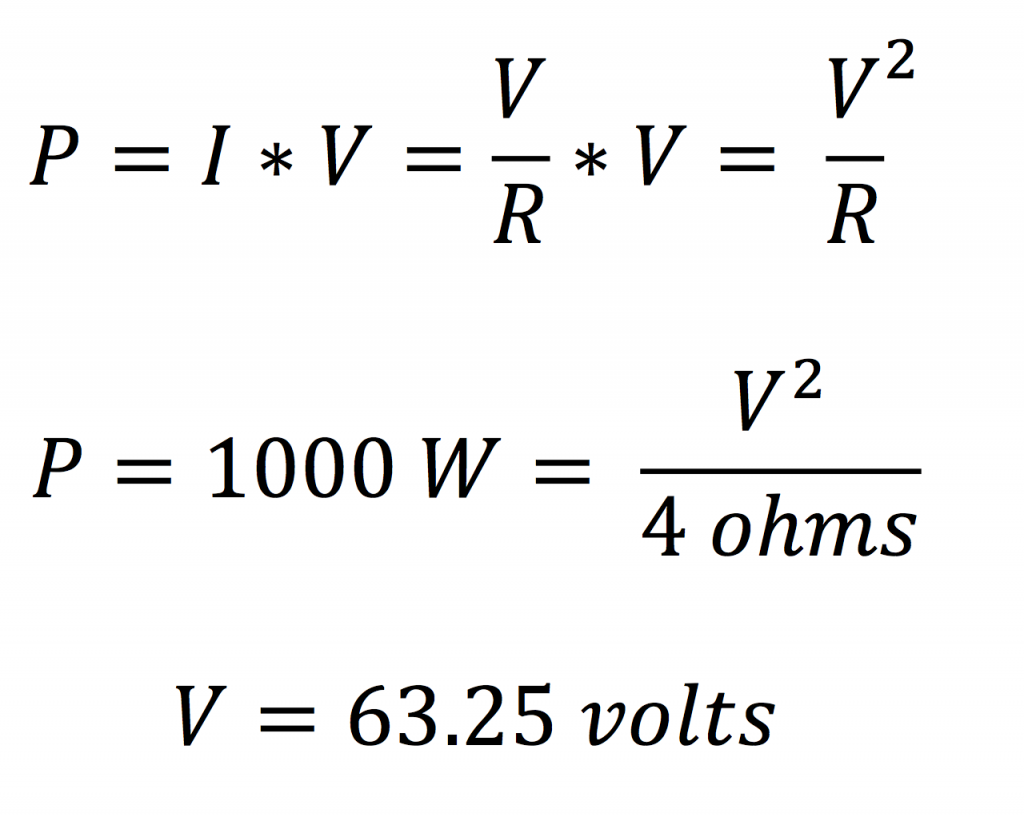Assuming this is the maximum voltage the power amp can produce, let’s see what happens when we decrease the resistance to 2 ohms.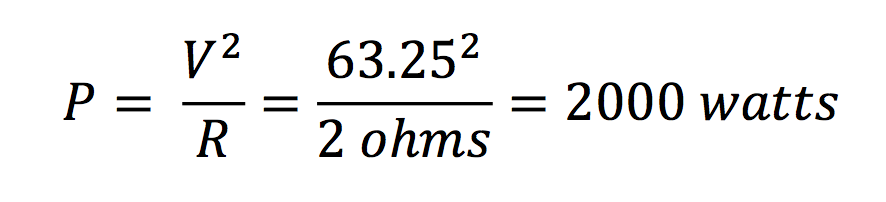By lowering the resistance of the speaker you’re asking the power amp to deliver much more energy per second than it is capable of delivering. In most power amps there’s a fuse to prevent disaster, but I’ve seen one or two power amps smoke and spark.

The use of the above equations assumes a simple, completely passive system. In reality the complexity of the systems would change the actual values from what we calculated above. Ohm’s Law works for passive circuit elements like the resistor. Passive means the resistor’s value of resistance does not change with different levels of voltage or current, nor does it add any energy to the system. While a power amp certainly has plenty of resistors in it, the operation of the amplifier cannot be fully analyzed with the above equations. We are making several assumptions and simplifications in order to get the general idea. For example, that same power amp rated at 1000 watts at 4 ohms is rated at 600 watts at 8 ohms (instead of 500 watts at 8 ohms). The overall concepts still hold true though and should give you a better understanding of the parameters listed with gear.

Well, because your amp is underpowered your sound guy had to turn it up to the max. He increased the gain so that the power amp was outputting the full 63.25 volts . Then you, assuming the resistances of all your monitors would add, actually connected them in parallel. Instead of increasing the total effective resistance of the monitors to 32 ohms, you actually decreased the resistance to 2. The combination of maximum voltage and low resistance resulted in: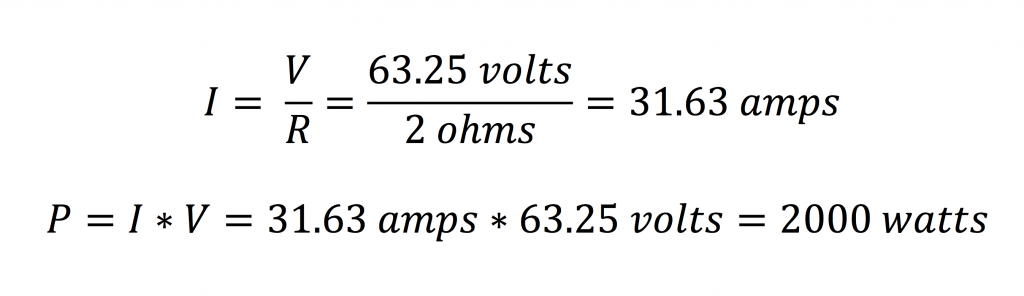I used the term “parallel” when describing the connection method that resulted in lowering the overall resistance of the monitors.

In the next article, we’ll dig deeper into series and parallel configurations as well as impedance matching.

### Missing our best stuff?

Sign up to be the first to learn about articles, videos, courses, freebies, giveaways, discounts & more. You'll also get a FREE gift today 🙌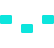/>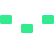/>/>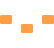/>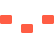/>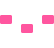/>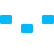/>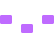/>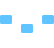/>/>